# How to Do Exponents in Python

The exponent of a number is the value we get by multiplying the number by itself a specific number of times. The Exponent of a number ‘x’ is written as xy and pronounced as “x raised to the power of y” where “x” is the base and “y” is the exponent. In this article, we’ll see how we can find the exponent of a number in python.

• By using the ** operator
• By using the pow() function
• By using the math module pow() function.
• By using the math.exp() function
• By using the NumPy.exp() function

## 1. By Using the ** Operator

The exponent of a number can be calculated by operator exponent (**) or power operator. This operator takes two operands and is placed between base and exponent.

### Example

```b = 7e = 3print("The exponent of positive number", b," is: ", b ** e)b = -7e = 3print("The exponent of negative number", b," is: ", b ** e)b = 7e = -3print("The negative exponent of positive number", b," is: ", b ** e)b = -7e = -3print("The negative exponent of negative number", b," is: ", b ** e)

```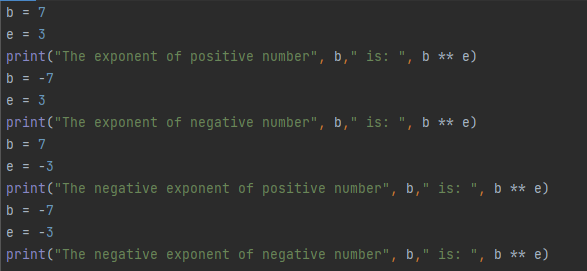### Output: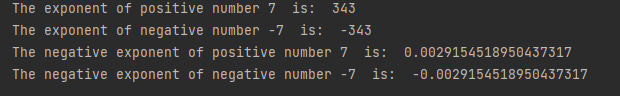In figure 1, ** operator takes two arguments base ‘b’ and exponent ‘e’ and get the output as shown in figure 2.

## 2. By Using the pow() Function

The built-in pow() function can also be used to find the exponent. It takes two arguments: base and power.

### Example

```print(pow(3,4))print(pow(-3,4))print(pow(3,-4))print(pow(-3,-4))

```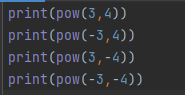### Output: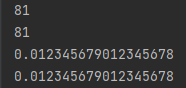In figure 3 we have used power function to find exponent of negative and positive numbers.

## 3. By Using the Math Module pow() Function

The math module also provides a power function that calculates the exponent of numbers. This function takes two arguments: base and exponent.

### Example

`import mathprint(math.pow(3,4))print(math.pow(-3,4))print(math.pow(3,-4))print(math.pow(-3,-4))`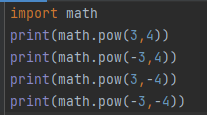### Output: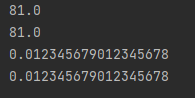In figure 5 we have used the power function of the math module to find the exponent of negative and positive numbers and get the result is a float point number as shown in figure 6.

## 4. By Using the math.exp() Function

The math module provides an exponent function that returns the float exponent of numbers. This function takes one argument: number. For the exponent function, the base is ‘E’ whose value is 2.718282.

### Example

`import mathprint(math.exp(3))print(math.exp(3.5))print(math.exp(-4))print(math.exp(-3.5))`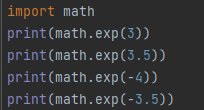### Output: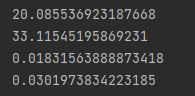In figure 7 we have used the exponent function of the math module to find the exponent of negative and positive numbers and get the result is a float point number as shown in figure 8.

## 5. By Using the NumPy.exp() Function

If we want to find out the exponent of more than one element then numpy.exp() is the best choice. Numpy module exp() method finds the exponent of the array elements.

### Example

`import numpy as nplist1 = np.array([4, 7.5, -1.5, 0.3, 12, 3.9, 6])result = np.exp(list1)print("exponent of list:", result)`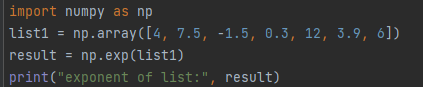### Output: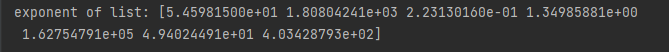In figure 9 we have imported numpy module. The numpy module exponent function can be used to find the exponent of the array and get output as shown in figure 10.